# Gaussian Processes

## Results

• Variational framework for learning inducing variables can be intepreted as minimizing a rigorously defined KL-divergence between the approximating and posterior processes. matthews15_spars_variat_method_kullb_leibl

## IMPORTANT

As of right now, must of the notes regarding this topic can be found in the notes for the book Guassian Proccesses for Machine Learning.

These will be moved in the future.

## Automatic Relevance Determination

Consider the covariance function: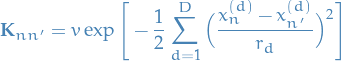1

The parameter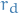is the length scale of the function along input dimension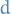. This implies that as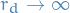the function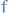varies less and less as a function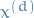, that is, the dth dimension becomes irrelevant.

Hence, given data, by learning the lengthscales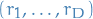is is possible to do automatic feature selection.

## Resources

• A Tutorial on Gaussian Processes (or why I don't use SVMs) by Zoubin Ghahramani A short presentation, providing an overview and showing how the objective function of a SVM is quite similar to a GP, but GP also has other nicer properties. He makes the following notes when comparing GPs with SVMs:
• GP incorporates uncertainty
• GP computes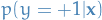, not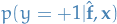as SVM
• GP can learn the kernel parameters automatically from data, no matter how flexible we make the kernel
• GP can learn the regularization parameter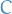without cross-validation
• Can combine automatic feature selection with learning using automatic relevance determination (ARD)

## Connection to RKHSs

• If both uses the same kernel, the posterior mean of a GP regression equals the estimator of kernel ridge regression

### Connections between GPs and Kernel Ridge Regression

#### Notation

•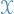non-empty set
•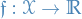be a function
• Given set of pairs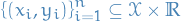for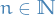• Assumption/model: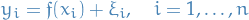where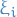is a zero-mean rv. which represents "noise" or uncontrollable error

• If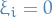for all,i .e. no output noise, then we call the problem interpolation
•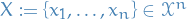•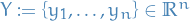•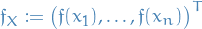in the noise-free/interpolation case

#### Gaussian Process Regression and Interpolation

• Also known as Kriging or Wiener-Kolmogorov prediction
• Non-parameter method for regression